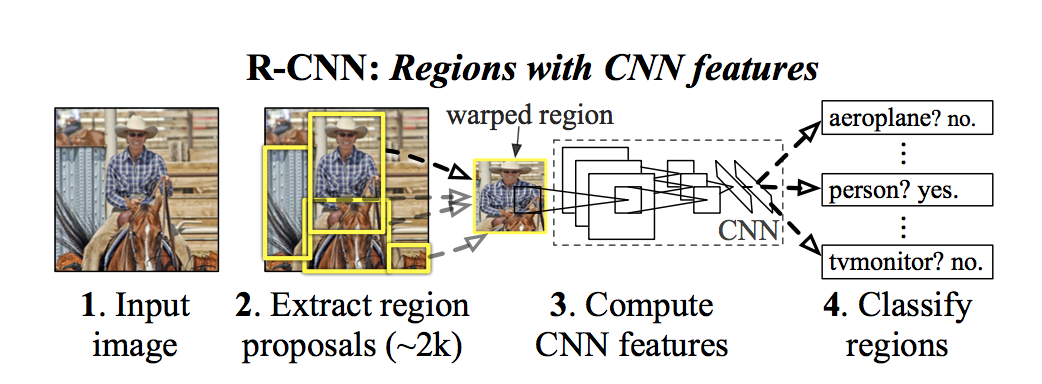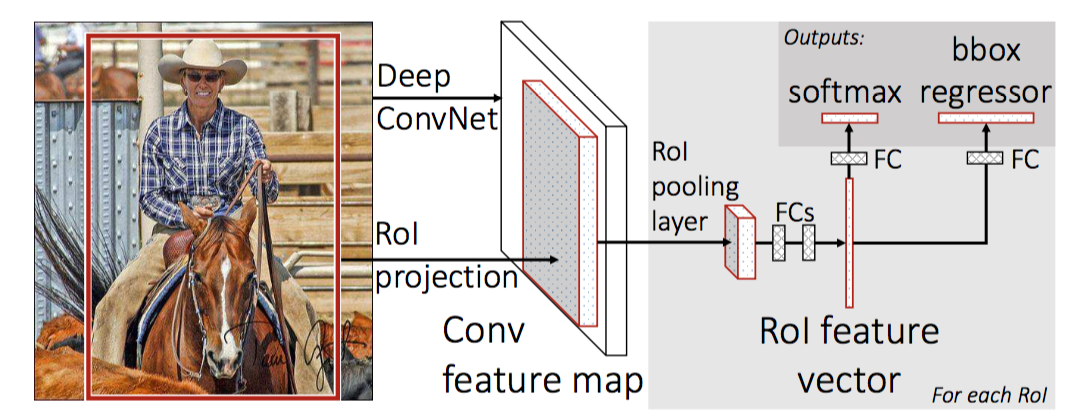R-CNN

Rich feature hierarchies for accurate object detection and semantic segmentation Tech report

Posted by GwanSiu on July 21, 2017

1. The Core Problem of Object detection

Given any image, object detection problem is to answer 2 questions: Where is the object? and What is the object?, which can be viewed as 2 subproblems in machine learning area: classification and regression. Hence, object detection consists of classifier and localizer in general. In detail, the pipeline of object detection is like that region proposal module—>feature extraction—>classifier(regressor)

In this article, I will try my best to summarize one of branch in deep learning structure for object detection That is the series of R-CNN.

2. R-CNN.

To my best knowledge, R-CNN is the first paper to apply deep learning model for object detection. The pipeline of R-CNN is shown below:RCNN In R-CNN, silding window methods is adopted for region proposal. Filters with different scale are used on each location. Then, proposed regions with different size are wrapped as the same size and then are feeded into the convolutional model, which plays a role of feature extraction. At the end, the classifer(regressor) is SVM, which is responsible for classifying object category and bounding box coordinate. SVM is the linear SVM for binary classification with hard sample mining strategy due to lots of negative samples.

For bounding box regression, series of R-CNN is adopted as:

$\begin{equation} t_{x}=(G_{x}-P_{x})/P_{w} \tag{3}\\ t_{y}=(G_{y}-P_{y})/P_{h}\\ t_{w}=\text{log}(G_{w}/P_{w})\\ t_{h}=\text{log}(G_{h}/P_{h})\\ \end{equation}$

CNN model achive state-of-the-art performence for feature extraction, compared with other shallow model, or other feature descriptor. RCNN take its advantage to extract more discriminative feature for object detection. Compared with Overfeat method(sliding windows), region proposal method adopted in R-CNN reduces candidate boxes in a great sense and shorter the inference time. Even thought, the inference time is not short enough because process of region proposal is time-consuming. In addition, warp operation distort image.

3. Fast RCNN - RoI Pooling Layer

There are several limitations in R-CNN:

1. R-CNN requires input image with fixed size(wrap operator).
2. R-CNN requires multi-stage training process. In ddetail, region proposal, feature extracture and clasiifier(regressor) are trained seperately.
3. The computational complexity and space complexity is high.
4. The inference time is long.

In order to solve some of these issues, Fast RCNN make 2 contributions:

1. Borrow the idea from SPPNet, RoI pooling layer is proposed in Fast R-CNN. It imposes no constraints on the size of input image. RoI pooling layer can be viewed as a special case of SPPNet, which is one spatial resolution level. With the advantage of RoI pooling layer, we can process image batch by batch.
2. The classifier of Fast RCNN adopt softmax function instead of SVM, so that it unify the process of feature extraction and classifier, and they can be jointly trained.
3. In order to reduce the computational complexity, SVD decomposition is used in the top of Fast RCNN. However, it can not solve high computational complexity and long inference time essencially.

The pipeline of Fast RCNN is shown below:4. Faster RCNN-RPN Network

The long inference time in Fast R-CNN is highly depended on the process of region proposal. Faster R-CNN consists of 3 parts: head, region proposal network and classifier. The first one is head, which is a base network pre-trained on the imagenet dataset. Region proposal network proposes region of interest, which is then extracted feature. Finally, it is the classifier.

Faster RCNN makes 2 contributions under the framework of RCNN:

1. To improve the speed, region proposal network is proposed to replace of the search selective methods and sliding windows methods under deep learning model for object detection. Compared with search selective method and sliding windows methods, region proposal network is more compatible with deep learning model because it directly predict bounding boxes coordinate from the feature map. Before RPN, search selective methods and sliding windows are two commom methods for region proposal.

2. Multi-scale prediction is another important issue in obejct detection system. In Faster RCNN, anchor mechanism is proposed, which can be viewed as a pecial case of sliding windows methods with 3 scales and 3 aspects. Before anchor, sliding windows and image pyramids are two main streams for multi-scale prediction. In detail, for sliding windows methods, we fixed the image size and use filers with different scales on each location of image to extract multi-scale feature. For image pyramids, we fixed the size of filter and use image pyramids to input image to extract multi-scale feature.The pipline of Faster R-CNN is shown below:More detail about how to train Faster RCNN, and structure detail. You can see one blog